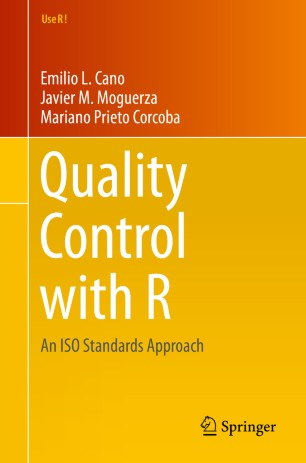Quality Control with R. An ISO Standards Approach (Cano EL, Moguerza JM and Prieto M, 2015).

# R packages

`qcc` seems to be the most popular and best maintained QC package for control charts.

``````suppressPackageStartupMessages(library(tidyverse))
# we use qcc 3.0 which is not released on CRAN yet
# devtools::install_github("luca-scr/qcc", build = TRUE, build_opts = c("--no-resave-data", "--no-manual"))
library(qcc)``````
``````## Package 'qcc' version 3.0
## Type 'citation("qcc")' for citing this R package in publications.``````
``````library(AcceptanceSampling)
library(SixSigma)
library(DiagrammeR)``````

# ISO standard QC Approach

ISO standards are defined by the International Organisation for Standardisation by technical committees using a process that is similar to peer-review. There are a lot of ISO standards covering Quality Control.

# QC tools

There are 7 general quality Ishikava tools, each of them is covered by numerous ISO documents.

• Cause and Effect Diagram
• Check sheet
• Control chart
• Histogram
• Pareto Chart
• Scatter Diagram
• Stratification
• Flow charts

## Cause and Effect Diagrams

The effect is usually undesired. As causes for a given effect for processes of the producing industry the ISO standard is 5Ms+E

• Manpower
• Materials
• Machines
• Methods
• Measurements
• Environment

• Manpower
• Events
• IT System
• Methods (Statistics, DevOps, Code base, Manual Processes)
• Measurements of Events (Data Entry, Data Coding)
• Environment (Culture, External Events, Regulations)

Specifically for the reporting of Adverse Drug Reactions in the form of individual case safety reports (ICSR) to Health Authorities (HA).

``````qcc::causeEffectDiagram(
cause = list(
Manpower = c(
"Medical Reviewer",
"Global Process Owner",
"Local Process Owner",
"IT Developer",
"Database Manager",
"Auditer"
),
Event = c(
"ICSR Quality",
"ICSR Detectability",
"ICSR Quantity",
"ICSR Complexity"
),
`IT Systems` = c(
"Availability",
"Complexity",
"Performance",
"Quality"
),
Methods = c(
"Medial Review Process\n(Data Guides)",
"Decisioning\n(Submission Rules)",
"Risk Management",
"Quality Mangement System",
"Change Management"
),
Measurements = c(
"Data Entry",
"Medical Coding"
),
Environment = c(
"HA Regulations",
"Local Cultures",
"Company Culture",
"Epidemiology",
"Local Health Systems"
)
),
effect = "ICSR not\nreported\nto HA"
)``````## Check Sheets

Serve to generate process related data, should be part of a general data collection plan. Will be automated for most processes.

• Audit Trail Data
• Quality Evidence
• IT Logs

Classically serves to attribute each effect (ICSR not reported to HA) to any of the suspected causes in the cause and effect diagram.

## Control Charts (Shewhart Charts)

Process Monitoring Charts, can be used to track whether process KPI are in or out of control.

``````data("pistonrings")
df <- as_tibble(pistonrings)

df_g1 <- df %>%
group_by(sample) %>%
mutate(rwn = row_number()) %>%
filter(rwn == 1)

qcc_shew <- qcc(
df\$diameter,
type = "xbar.one",
rules = c(1, 2, 3, 4)
)

qcc_shew``````
``````## ── Quality Control Chart ─────────────────────────
##
## Chart type                 = xbar.one
## Data (phase I)             = df\$diameter
## Number of groups           = 200
## Group sample size          = 1
## Center of group statistics = 74.0036
## Standard deviation         = 0.01001461
##
## Control limits at nsigmas  = 3
##       LCL      UCL
##  73.97356 74.03365``````
``str(qcc_shew)``
``````## List of 12
##  \$ call      : language qcc(data = df\$diameter, type = "xbar.one", rules = c(1, 2, 3, 4))
##  \$ type      : chr "xbar.one"
##  \$ data.name : chr "df\$diameter"
##  \$ data      : num [1:200, 1] 74 74 74 74 74 ...
##   ..- attr(*, "dimnames")=List of 2
##   .. ..\$ Group  : chr [1:200] "1" "2" "3" "4" ...
##   .. ..\$ Samples: NULL
##  \$ statistics: Named num [1:200] 74 74 74 74 74 ...
##   ..- attr(*, "names")= chr [1:200] "1" "2" "3" "4" ...
##  \$ sizes     : int [1:200] 1 1 1 1 1 1 1 1 1 1 ...
##  \$ center    : num 74
##  \$ std.dev   : num 0.01
##  \$ rules     : num [1:4] 1 2 3 4
##  \$ nsigmas   : num 3
##  \$ limits    : num [1, 1:2] 74 74
##   ..- attr(*, "dimnames")=List of 2
##   .. ..\$ : chr ""
##   .. ..\$ : chr [1:2] "LCL" "UCL"
##  \$ violations: num [1:200] NA NA NA NA NA NA NA NA NA NA ...
##   ..- attr(*, "WesternElectricRules")= num [1:4] 1 2 3 4
##  - attr(*, "class")= chr "qcc"``````
``plot(qcc_shew)``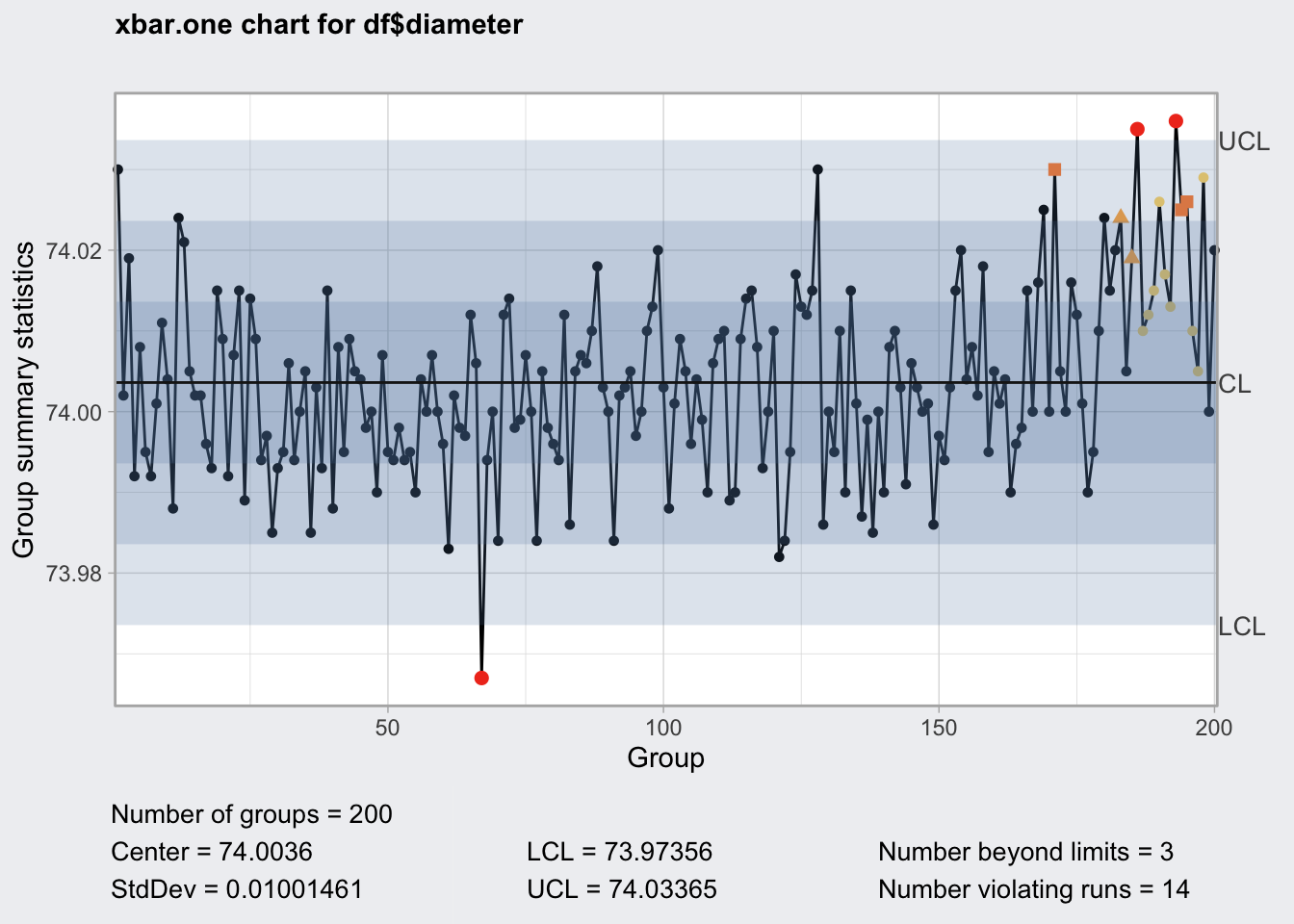``qcc_shew\$violations``
``````##    NA NA NA NA NA NA NA NA NA NA NA NA NA NA NA NA NA NA NA NA NA NA NA NA NA
##   NA NA NA NA NA NA NA NA NA NA NA NA NA NA NA NA NA NA NA NA NA NA NA NA NA
##   NA NA NA NA NA NA NA NA NA NA NA NA NA NA NA NA  1 NA NA NA NA NA NA NA NA
##   NA NA NA NA NA NA NA NA NA NA NA NA NA NA NA NA NA NA NA NA NA NA NA NA NA
##  NA NA NA NA NA NA NA NA NA NA NA NA NA NA NA NA NA NA NA NA NA NA NA NA NA
##  NA NA NA NA NA NA NA NA NA NA NA NA NA NA NA NA NA NA NA NA NA NA NA NA NA
##  NA NA NA NA NA NA NA NA NA NA NA NA NA NA NA NA NA NA NA NA  2 NA NA NA NA
##  NA NA NA NA NA NA NA  3 NA  3  1  4  4  4  4  4  4  1  2  2  4  4  4 NA NA
## attr(,"WesternElectricRules")
##  1 2 3 4``````
``qcc_shew\$rules``
``##  1 2 3 4``

`qcc` uses the Western Electric Rules to flag out of control values.

• One point plots outside 3-sigma control limits.
• Two of three consecutive points plot beyond a 2-sigma limit.
• Four of five consecutive points plot beyond a 1-sigma limit.
• Eight consecutive points plot on one side of the center line.

These rules do not appear to be customizable, it seems to me that Nelson Rules are a bit more complete and cover more non-random scenarios such as trends and oscillation.

The control chart type depends on the data type and its distribution. There is a decision tree for the chart selection.

``````DiagrammeR::mermaid(
"graph TB
A(Select Control Chart)-->B{data type}
B --> |Discrete| C{Distribution}
B --> |Continuous| D{Sample Size}
C --> |Poisson| E{Sample Size}
C --> |Binominal| F{Frequency}
E --> |constant| G[c-chart]
E --> |variable| H[u-chart]
F --> |count| I[np-chart]
F --> |proportion| J[p-chart]
D --> |n = 1| K[x.one + R chart]
D --> |n = 2-10| L[x + R chart]
D --> |n > 10| M[x + S chart]
"
)``````

## Histogram

self explanatory, explore distribution of continuous variable.

``````ggplot(df_g1) +
geom_histogram(aes(diameter))``````
``## `stat_bin()` using `bins = 30`. Pick better value with `binwidth`.``## Pareto Chart

Plot Frequency of effect causes, apply 80:20 rule

``````defect <- c(80, 27, 66, 94, 33)
names(defect) <- c("HA Regulations", "Risk Management", "Data Entry", "Change Management", "IT System Performance")
qcc_pareto <- qcc::paretoChart(defect)
str(qcc_pareto)``````
``````## List of 3
##  \$ call     : language qcc::paretoChart(data = defect)
##  \$ data.name: chr "defect"
##  \$ tab      : num [1:5, 1:4] 94 80 66 33 27 94 174 240 273 300 ...
##   ..- attr(*, "dimnames")=List of 2
##   .. ..\$ defect: chr [1:5] "Change Management" "HA Regulations" "Data Entry" "IT System Performance" ...
##   .. ..\$       : chr [1:4] "Frequency" "Cum.Freq." "Percentage" "Cum.Percent."
##  - attr(*, "class")= chr "paretoChart"``````
``````plot(qcc_pareto) +
theme(axis.text.x = element_text(angle = 90))``````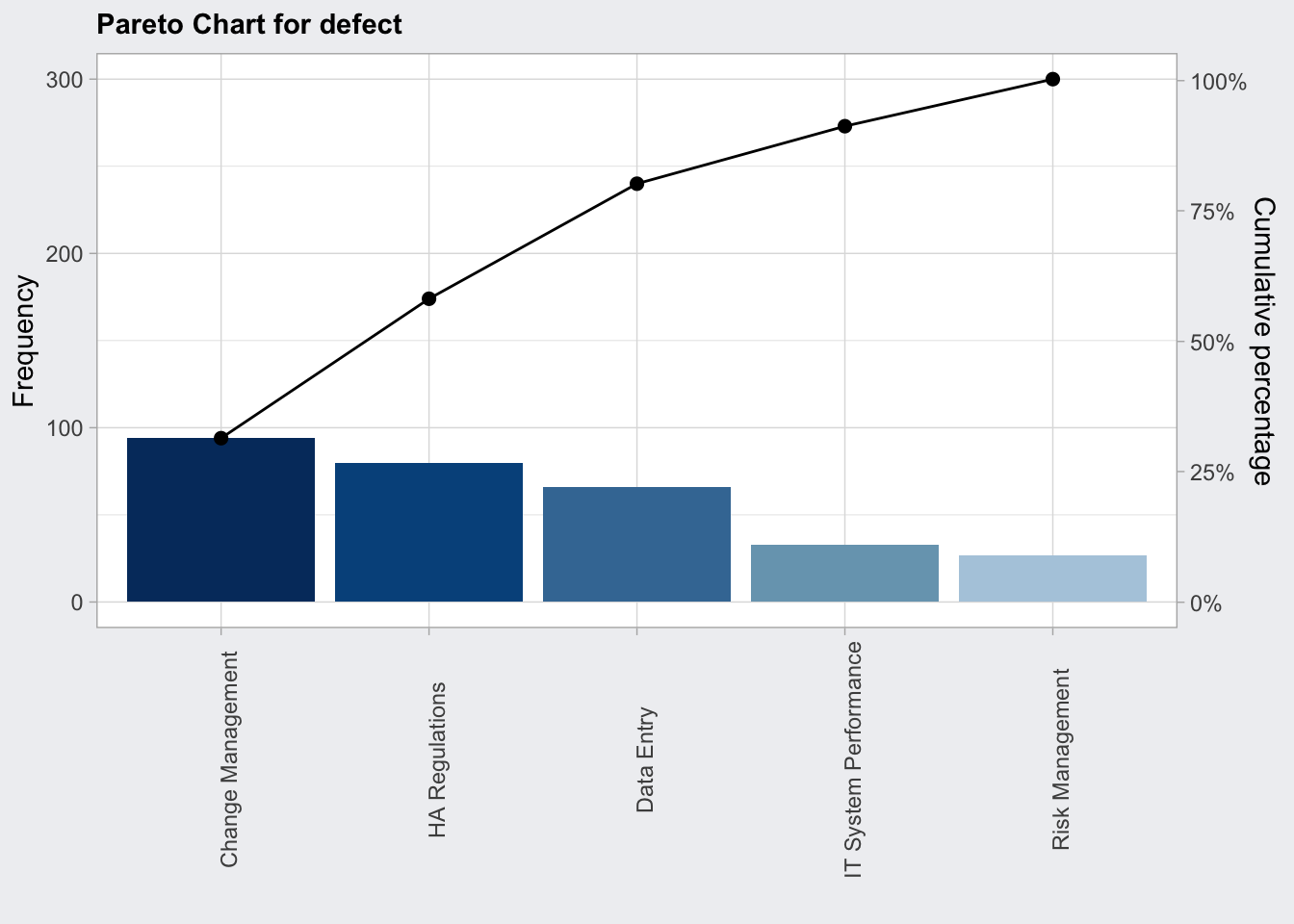## Scatter Plots

• validate established cause and effect relationships
• multivariate control diagrams

## Stratification

• identify relevant process subgroups by which to stratify the analysis
• use of process flow charts

# Control Charts

• ideally have even sample sizes to obtain even upper and lower control limits, although `qcc` allow you to create control charts with uneven sample sizes but oc plots cannot be created, thus it is difficult to make good estimates about appropriate sample sizes.
• plot in pairs to monitor different summary statistics like x-bar chart (means) and range chart (R chart) as a measure for variance
• use Shewart’s formula to estimate control limits
• phase I determine a calibration period during which the process is in control and determine control limits
• phase II monitor process for out of range values

## Continuous Grouped Variables

We are going to measure a continuous variable over time such as the thickness of a board.

Controls Charts use the population standard deviation to flag out of control data points.

Determine population standard deviation:

• determine a calibration period during which the process is in control (set n)
• at each time point measure samples and take the mean
• according to the central limit theorem the distribution of the means will be normally distributed
• measure population standard deviation of the means `sqrt((n-1)/n) * sd(x)`
• in statistical process control this is considered to be biased
• instead the range and the shewart constant (d2/c4) which depends on the number of measurements (n) are used by the Shewart’s formula to estimate control limits

Determine sample size: - ARL is the average run length (number of consecutive samples) needed to get a point that is outside the upper and lower control limits. For a normal distribution the ARL is 370. So every 370 measurements we will get a false positive signal. - The power of a control chart depends on the sample size. The operator characteristics (OC) plots the probabilty of a false negative (type two error) against the process shift in standard deviations for several sample sizes (n). For a given shift in process deviation we can also determine the probability beta for a false negative using the OC curve. - ARL = 1 / (1 - beta)

### X-bar Chart

• Monitors the mean
• uses d2 Shewart’s constants
``df``
``````## # A tibble: 200 x 3
##    diameter sample trial
##       <dbl>  <int> <lgl>
##  1     74.0      1 TRUE
##  2     74.0      1 TRUE
##  3     74.0      1 TRUE
##  4     74.0      1 TRUE
##  5     74.0      1 TRUE
##  6     74.0      2 TRUE
##  7     74.0      2 TRUE
##  8     74.0      2 TRUE
##  9     74.0      2 TRUE
## 10     74.0      2 TRUE
## # … with 190 more rows``````
``````# pivot from long to wide
qcc_gr <- qcc::qccGroups(df, diameter, sample)

qcc_gr``````
``````##      [,1]   [,2]   [,3]   [,4]   [,5]
## 1  74.030 74.002 74.019 73.992 74.008
## 2  73.995 73.992 74.001 74.011 74.004
## 3  73.988 74.024 74.021 74.005 74.002
## 4  74.002 73.996 73.993 74.015 74.009
## 5  73.992 74.007 74.015 73.989 74.014
## 6  74.009 73.994 73.997 73.985 73.993
## 7  73.995 74.006 73.994 74.000 74.005
## 8  73.985 74.003 73.993 74.015 73.988
## 9  74.008 73.995 74.009 74.005 74.004
## 10 73.998 74.000 73.990 74.007 73.995
## 11 73.994 73.998 73.994 73.995 73.990
## 12 74.004 74.000 74.007 74.000 73.996
## 13 73.983 74.002 73.998 73.997 74.012
## 14 74.006 73.967 73.994 74.000 73.984
## 15 74.012 74.014 73.998 73.999 74.007
## 16 74.000 73.984 74.005 73.998 73.996
## 17 73.994 74.012 73.986 74.005 74.007
## 18 74.006 74.010 74.018 74.003 74.000
## 19 73.984 74.002 74.003 74.005 73.997
## 20 74.000 74.010 74.013 74.020 74.003
## 21 73.988 74.001 74.009 74.005 73.996
## 22 74.004 73.999 73.990 74.006 74.009
## 23 74.010 73.989 73.990 74.009 74.014
## 24 74.015 74.008 73.993 74.000 74.010
## 25 73.982 73.984 73.995 74.017 74.013
## 26 74.012 74.015 74.030 73.986 74.000
## 27 73.995 74.010 73.990 74.015 74.001
## 28 73.987 73.999 73.985 74.000 73.990
## 29 74.008 74.010 74.003 73.991 74.006
## 30 74.003 74.000 74.001 73.986 73.997
## 31 73.994 74.003 74.015 74.020 74.004
## 32 74.008 74.002 74.018 73.995 74.005
## 33 74.001 74.004 73.990 73.996 73.998
## 34 74.015 74.000 74.016 74.025 74.000
## 35 74.030 74.005 74.000 74.016 74.012
## 36 74.001 73.990 73.995 74.010 74.024
## 37 74.015 74.020 74.024 74.005 74.019
## 38 74.035 74.010 74.012 74.015 74.026
## 39 74.017 74.013 74.036 74.025 74.026
## 40 74.010 74.005 74.029 74.000 74.020``````
``````q1 <- qcc(
qcc_gr[1:25, ],
type = "xbar",
newdata = qcc_gr[26:nrow(qcc_gr), ],
rules = c(1, 2, 3, 4)
)

q1``````
``````## ── Quality Control Chart ─────────────────────────
##
## Chart type                 = xbar
## Data (phase I)             = qcc_gr[1:25, ]
## Number of groups           = 25
## Group sample size          = 5
## Center of group statistics = 74.00118
## Standard deviation         = 0.009785039
##
## New data (phase II)        = qcc_gr[26:nrow(qcc_gr), ]
## Number of groups           = 15
## Group sample size          = 5
##
## Control limits at nsigmas  = 3
##       LCL     UCL
##  73.98805 74.0143``````
``plot(q1)````````q1_oc <- qcc::ocCurves(q1)

q1_oc``````
``````## ── Operating Characteristic Curves ───────────────
##
## Chart type                 = xbar
## Prob. type II error (beta) =
##               sample size
## shift (StdDev)      1      5     10     15     20
##            0.0 0.9973 0.9973 0.9973 0.9973 0.9973
##            0.1 0.9972 0.9966 0.9959 0.9952 0.9944
##            0.2 0.9968 0.9944 0.9909 0.9869 0.9823
##            :
##            4.9 0.0287 0.0000 0.0000 0.0000 0.0000
##            5.0 0.0228 0.0000 0.0000 0.0000 0.0000
## Average run length (ARL)   =
##               sample size
## shift (StdDev)   1   5  10  15  20
##            0.0 370 370 370 370 370
##            0.1 353 296 244 206 178
##            0.2 308 178 110  76  57
##            :
##            4.9   1   1   1   1   1
##            5.0   1   1   1   1   1``````
``plot(q1_oc)````````arl_n5 <- q1_oc\$ARL %>%
as_tibble(rownames = "shift_stdev") %>%
filter(shift_stdev == "1.0") %>%
pull(`5`)

arl_n5``````
``##  4.495312``

So for a change in average diameter by 0.009785 we need in average 4.4953122 runs to detect a change in the mean statistic using 5 samples.

### R Chart

• monitors process stability by means of the sample ranges
• uses d3 and d2 Shewart’s constants
• appropriate for sample sizes < 8
``````q2 <- qcc(
qcc_gr[1:25, ],
type = "R",
newdata = qcc_gr[26:nrow(qcc_gr), ],
rules = c(1, 2, 3, 4)
)

q2``````
``````## ── Quality Control Chart ─────────────────────────
##
## Chart type                 = R
## Data (phase I)             = qcc_gr[1:25, ]
## Number of groups           = 25
## Group sample size          = 5
## Center of group statistics = 0.02276
## Standard deviation         = 0.009785039
##
## New data (phase II)        = qcc_gr[26:nrow(qcc_gr), ]
## Number of groups           = 15
## Group sample size          = 5
##
## Control limits at nsigmas  = 3
##  LCL        UCL
##    0 0.04812533``````
``plot(q2)``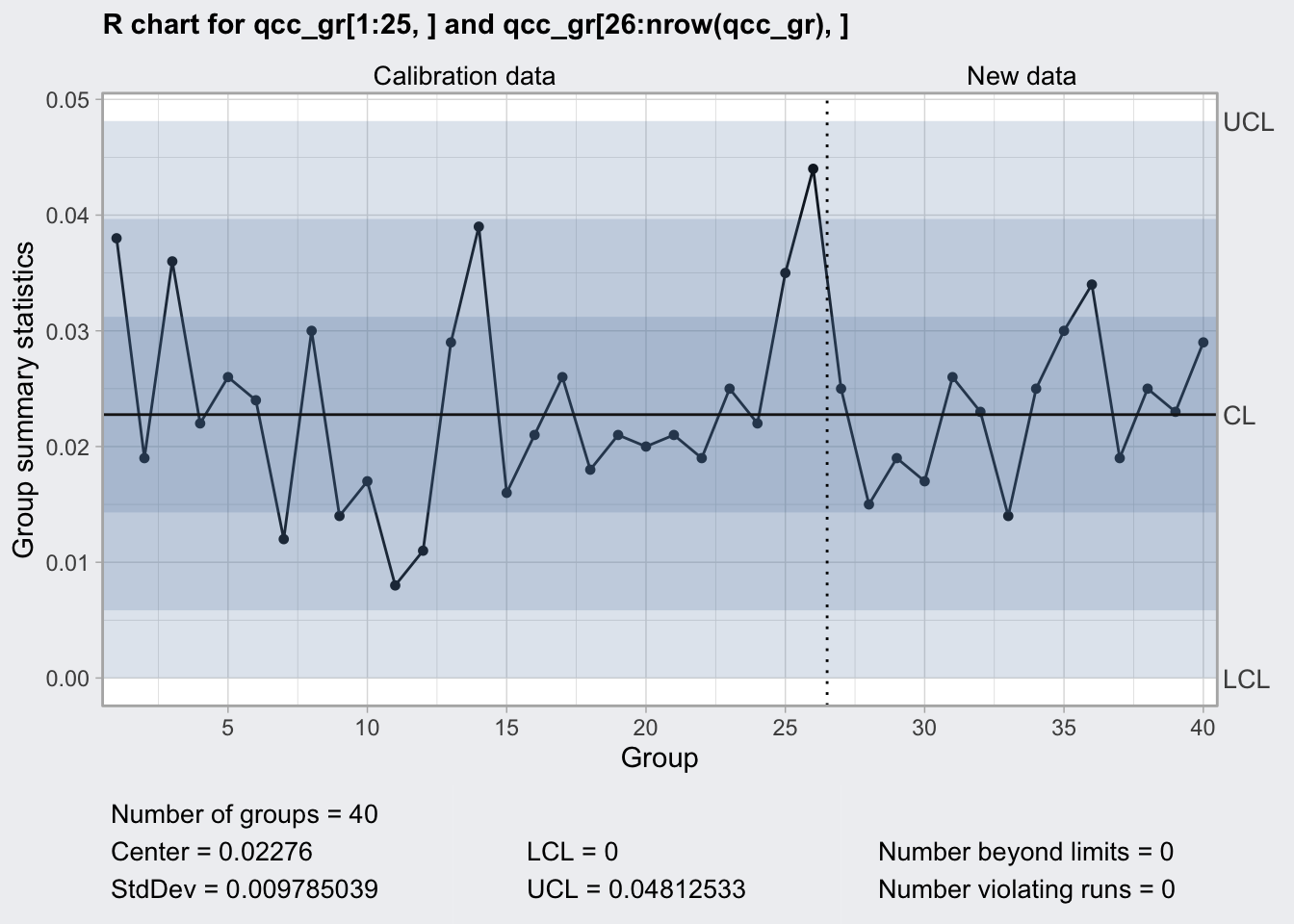``````q2_oc <- qcc::ocCurves(q2)

# throws error, https://github.com/luca-scr/qcc/issues/31
# plot(q2_oc)

q2_oc``````
``````## ── Operating Characteristic Curves ───────────────
##
## Chart type                 = R
## Prob. type II error (beta) =
##                 sample size
## scale multiplier      2      5     10     15     20
##              1.0 0.9908 0.9954 0.9956 0.9955 0.9954
##              1.1 0.9822 0.9864 0.9841 0.9818 0.9798
##              1.2 0.9701 0.9692 0.9583 0.9486 0.9403
##              :
##              5.9 0.3413 0.0233 0.0003 0.0000 0.0000
##              6.0 0.3360 0.0219 0.0002 0.0000 0.0000
## Average run length (ARL)   =
##                 sample size
## scale multiplier   2   5  10  15  20
##              1.0 109 217 229 223 217
##              1.1  56  74  63  55  50
##              1.2  33  32  24  19  17
##              :
##              5.9   2   1   1   1   1
##              6.0   2   1   1   1   1``````
``````arl_n5 <- q2_oc\$ARL %>%
as_tibble(rownames = "scale_multiplier") %>%
filter(scale_multiplier == "1.5") %>%
pull(`5`)

arl_n5``````
``##  7.198125``

So for a change in range by 50% need in average 7.1981249 runs using 5 samples.

### S Chart

• monitors process stability by means of standard deviations
• uses c4 Shewart’s constant
• appropriate for sample sizes >= 8
``````q3 <- qcc(
qcc_gr[1:25, ],
type = "S",
newdata = qcc_gr[26:nrow(qcc_gr), ],
rules = c(1, 2, 3, 4)
)

q3``````
``````## ── Quality Control Chart ─────────────────────────
##
## Chart type                 = S
## Data (phase I)             = qcc_gr[1:25, ]
## Number of groups           = 25
## Group sample size          = 5
## Center of group statistics = 0.009240037
## Standard deviation         = 0.009829977
##
## New data (phase II)        = qcc_gr[26:nrow(qcc_gr), ]
## Number of groups           = 15
## Group sample size          = 5
##
## Control limits at nsigmas  = 3
##  LCL        UCL
##    0 0.01930242``````
``plot(q3)``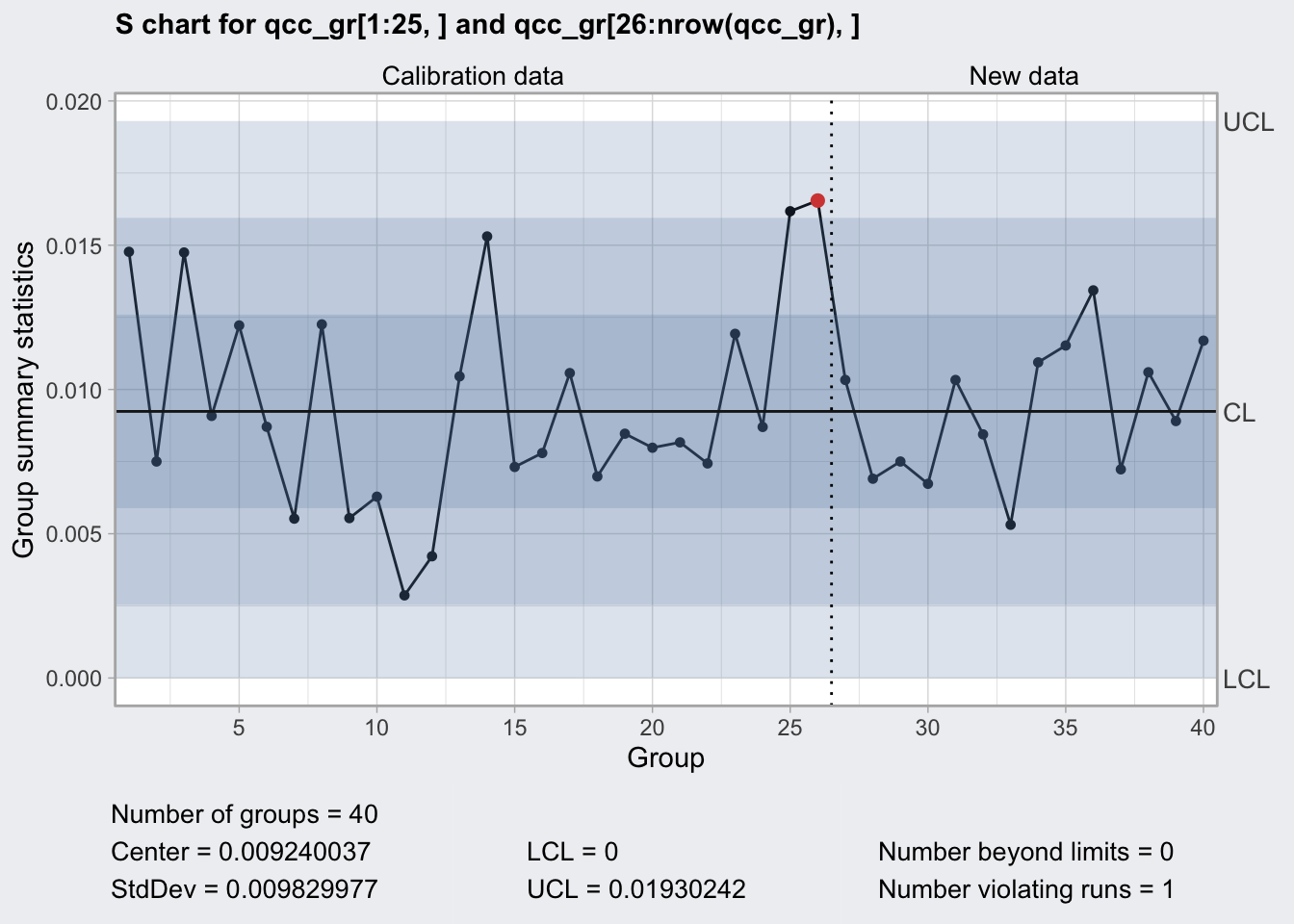``````q3_oc <- qcc::ocCurves(q3)

# throws error, https://github.com/luca-scr/qcc/issues/31
# plot(q3_oc)

q3_oc``````
``````## ── Operating Characteristic Curves ───────────────
##
## Chart type                 = S
## Prob. type II error (beta) =
##                 sample size
## scale multiplier      2      5     10     15     20
##              1.0 0.9908 0.9961 0.9970 0.9972 0.9972
##              1.1 0.9822 0.9874 0.9860 0.9838 0.9812
##              1.2 0.9701 0.9700 0.9574 0.9425 0.9261
##              :
##              5.9 0.3413 0.0212 0.0001 0.0000 0.0000
##              6.0 0.3360 0.0199 0.0001 0.0000 0.0000
## Average run length (ARL)   =
##                 sample size
## scale multiplier   2   5  10  15  20
##              1.0 109 256 333 351 358
##              1.1  56  79  72  62  53
##              1.2  33  33  23  17  14
##              :
##              5.9   2   1   1   1   1
##              6.0   2   1   1   1   1``````
``````arl_n5 <- q3_oc\$ARL %>%
as_tibble(rownames = "scale_multiplier") %>%
filter(scale_multiplier == "1.5") %>%
pull(`5`)``````

So for a change in range by 50% need in average 6.9559271 runs using 5 samples.

## Continuous Non-Grouped Variables

If a process is described best by a continuous one at a time measurement we cannot use sampling

• central limit theorem does not apply
• normality needs to be confirmed
• if possible use power transformation to normalize
• accompany x-bar chart with moving range chart

### X-bar chart

• uses the moving range to calculate global standard deviation
• takes Shewart’s constant d2 for n = 2
``````# airplane paint viscosity, one batch takes hours to produce and no rational subgroups can be formed
data("viscosity")
df_vis <- viscosity

df_vis``````
``````##    batch viscosity trial
## 1      1     34.05  TRUE
## 2      2     34.40  TRUE
## 3      3     33.59  TRUE
## 4      4     35.96  TRUE
## 5      5     34.70  TRUE
## 6      6     33.51  TRUE
## 7      7     33.79  TRUE
## 8      8     34.04  TRUE
## 9      9     34.52  TRUE
## 10    10     33.75  TRUE
## 11    11     33.27  TRUE
## 12    12     33.71  TRUE
## 13    13     34.03  TRUE
## 14    14     34.58  TRUE
## 15    15     34.02  TRUE
## 16    16     33.97  TRUE
## 17    17     34.05  TRUE
## 18    18     34.04  TRUE
## 19    19     33.73  TRUE
## 20    20     34.05  TRUE
## 21    21     34.39 FALSE
## 22    22     33.75 FALSE
## 23    23     33.40 FALSE
## 24    24     33.27 FALSE
## 25    25     34.65 FALSE
## 26    26     34.80 FALSE
## 27    27     34.55 FALSE
## 28    28     35.40 FALSE
## 29    29     34.75 FALSE
## 30    30     34.50 FALSE
## 31    31     34.70 FALSE
## 32    32     34.29 FALSE
## 33    33     34.61 FALSE
## 34    34     34.49 FALSE
## 35    35     35.03 FALSE``````
``````q4 <- qcc(
df_vis\$viscosity[df_vis\$trial],
type = "xbar.one",
newdata = df_vis\$viscosity[! df_vis\$trial],
rules = c(1, 2, 3, 4)
)

q4``````
``````## ── Quality Control Chart ─────────────────────────
##
## Chart type                 = xbar.one
## Data (phase I)             = df_vis\$viscosity[df_vis\$trial]
## Number of groups           = 20
## Group sample size          = 1
## Center of group statistics = 34.088
## Standard deviation         = 0.5076521
##
## New data (phase II)        = df_vis\$viscosity[!df_vis\$trial]
## Number of groups           = 15
## Group sample size          = 1
##
## Control limits at nsigmas  = 3
##       LCL      UCL
##  32.56504 35.61096``````
``plot(q4)``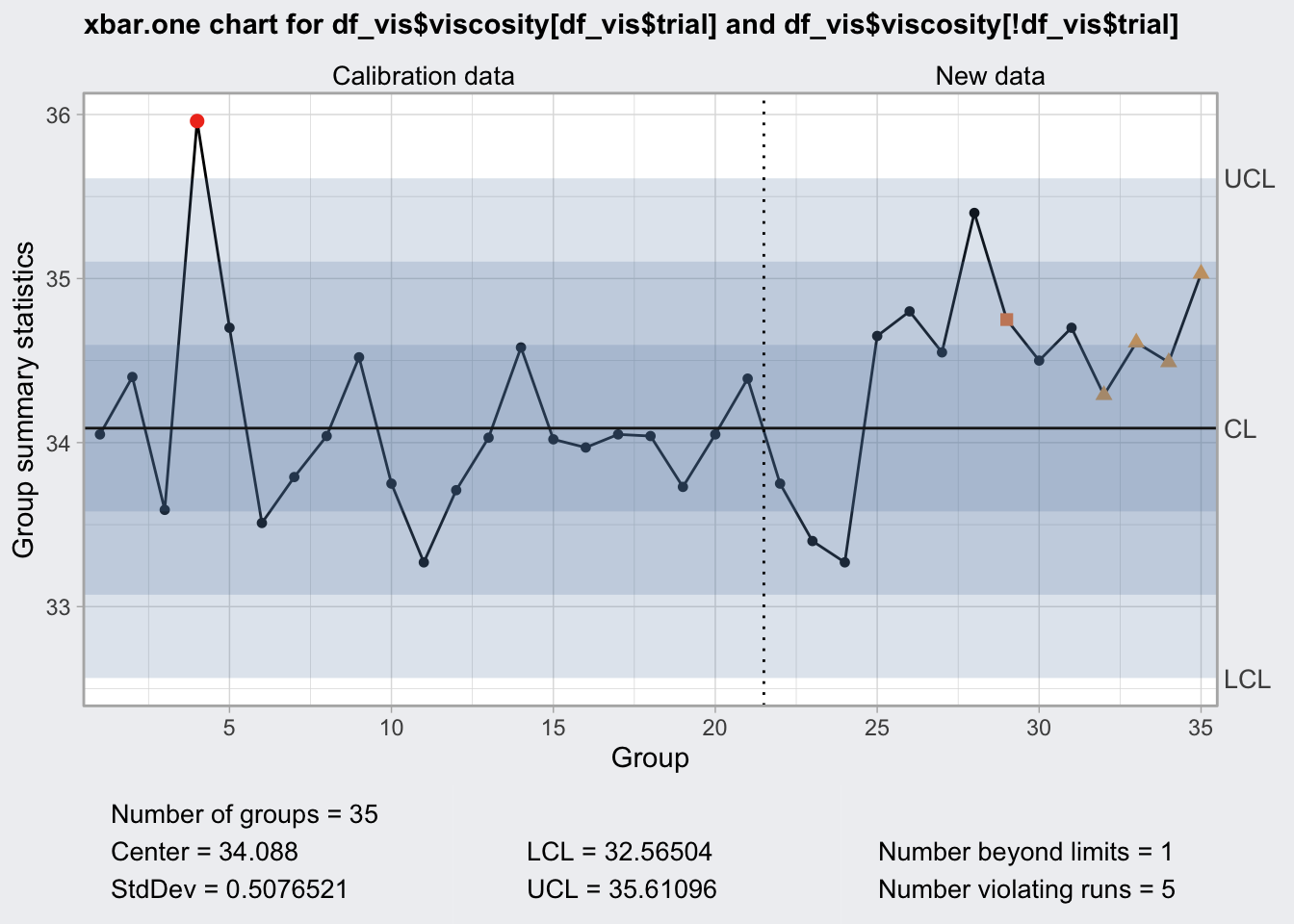### R Chart

• uses the range between a given and the next measurement
``````vis_mat <- df_vis %>%
as.matrix()

q5 <- qcc(
vis_mat[df_vis\$trial[1:nrow(df_vis)-1], ],
type = "R",
newdata = vis_mat[! df_vis\$trial[1:nrow(df_vis)-1], ]
)

q5``````
``````## ── Quality Control Chart ─────────────────────────
##
## Chart type                 = R
## Data (phase I)             = vis_mat[df_vis\$trial[1:nrow(df_vis) - 1], ]
## Number of groups           = 20
## Group sample size          = 2
## Center of group statistics = 0.561
## Standard deviation         = 0.4973404
##
## New data (phase II)        = vis_mat[!df_vis\$trial[1:nrow(df_vis) - 1], ]
## Number of groups           = 14
## Group sample size          = 2
##
## Control limits at nsigmas  = 3
##  LCL      UCL
##    0 1.832953``````
``plot(q5)``## Discrete Measurements

### p and np Charts

• measure frequency or relative frequency
• p is for relative frequency
• np for frequency
``````data("orangejuice")
df_oj <- orangejuice

q6 <- qcc(
df_oj\$D[df_oj\$trial],
df_oj\$size[df_oj\$trial],
type = "p",
newdata = df_oj\$D[! df_oj\$trial],
newsizes = df_oj\$size[! df_oj\$trial],
rules = c(1, 2, 3, 4)
)

q6``````
``````## ── Quality Control Chart ─────────────────────────
##
## Chart type                 = p
## Data (phase I)             = df_oj\$D[df_oj\$trial]
## Number of groups           = 30
## Group sample size          = 50
## Center of group statistics = 0.2313333
## Standard deviation         = 0.05963526
##
## New data (phase II)        = df_oj\$D[!df_oj\$trial]
## Number of groups           = 24
## Group sample size          = 50
##
## Control limits at nsigmas  = 3
##          LCL       UCL
##   0.05242755 0.4102391
##   0.05242755 0.4102391
## :
##   0.05242755 0.4102391``````
``plot(q6)````````q6_oc <- qcc::ocCurves(q6)

q6_oc``````
``````## ── Operating Characteristic Curves ───────────────
##
## Chart type                 = p
## Prob. type II error (beta) =
##                       
## fraction nonconforming   [,1]
##                   0.00 0.0000
##                   0.01 0.0894
##                   0.02 0.2642
##                   :
##                   0.99 0.0000
##                   1.00 0.0000
## Average run length (ARL)   =
##                       
## fraction nonconforming [,1]
##                   0.00    1
##                   0.01    1
##                   0.02    1
##                   :
##                   0.99    1
##                   1.00    1``````
``plot(q6_oc)``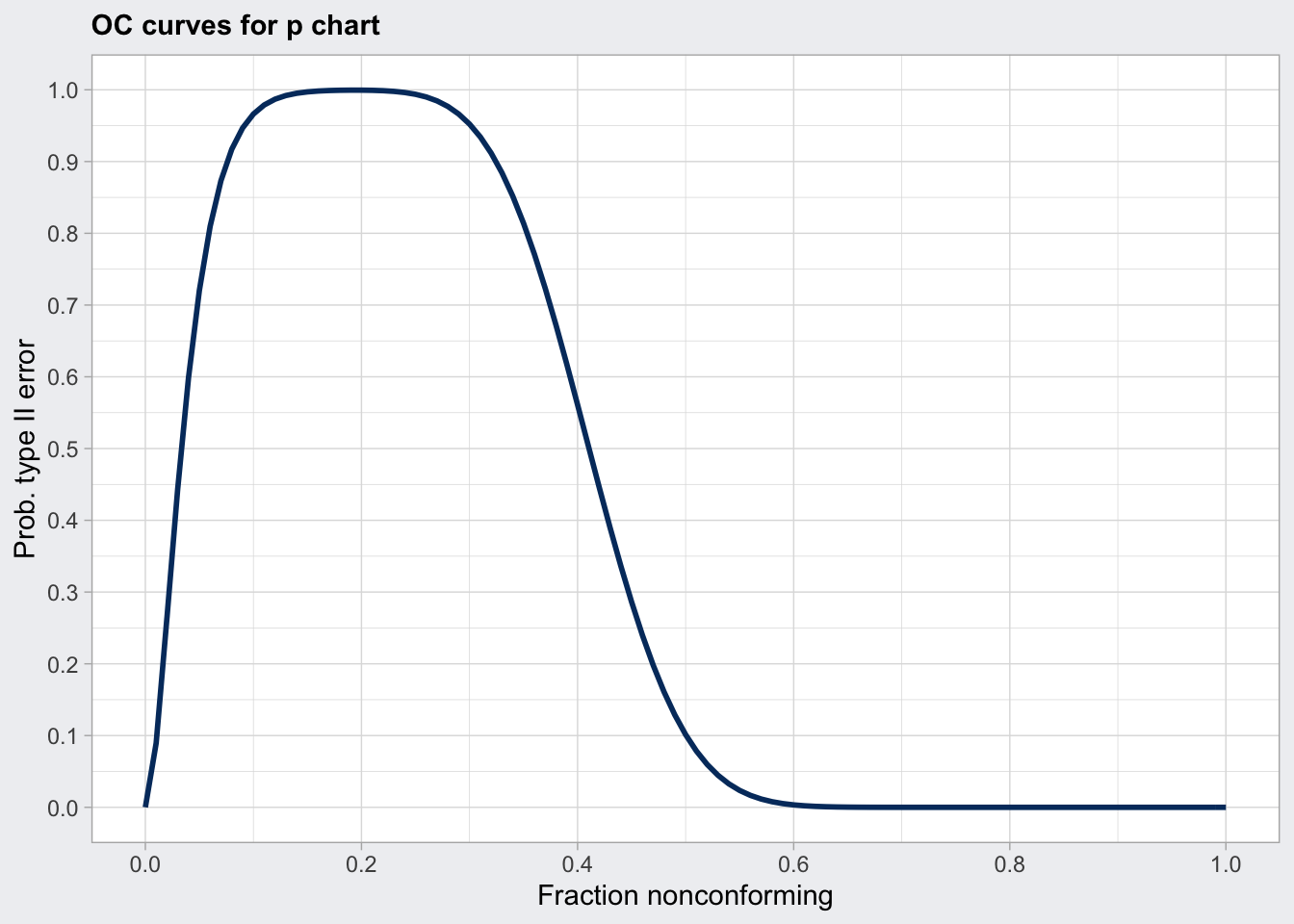``plot(q6_oc, what = "ARL")``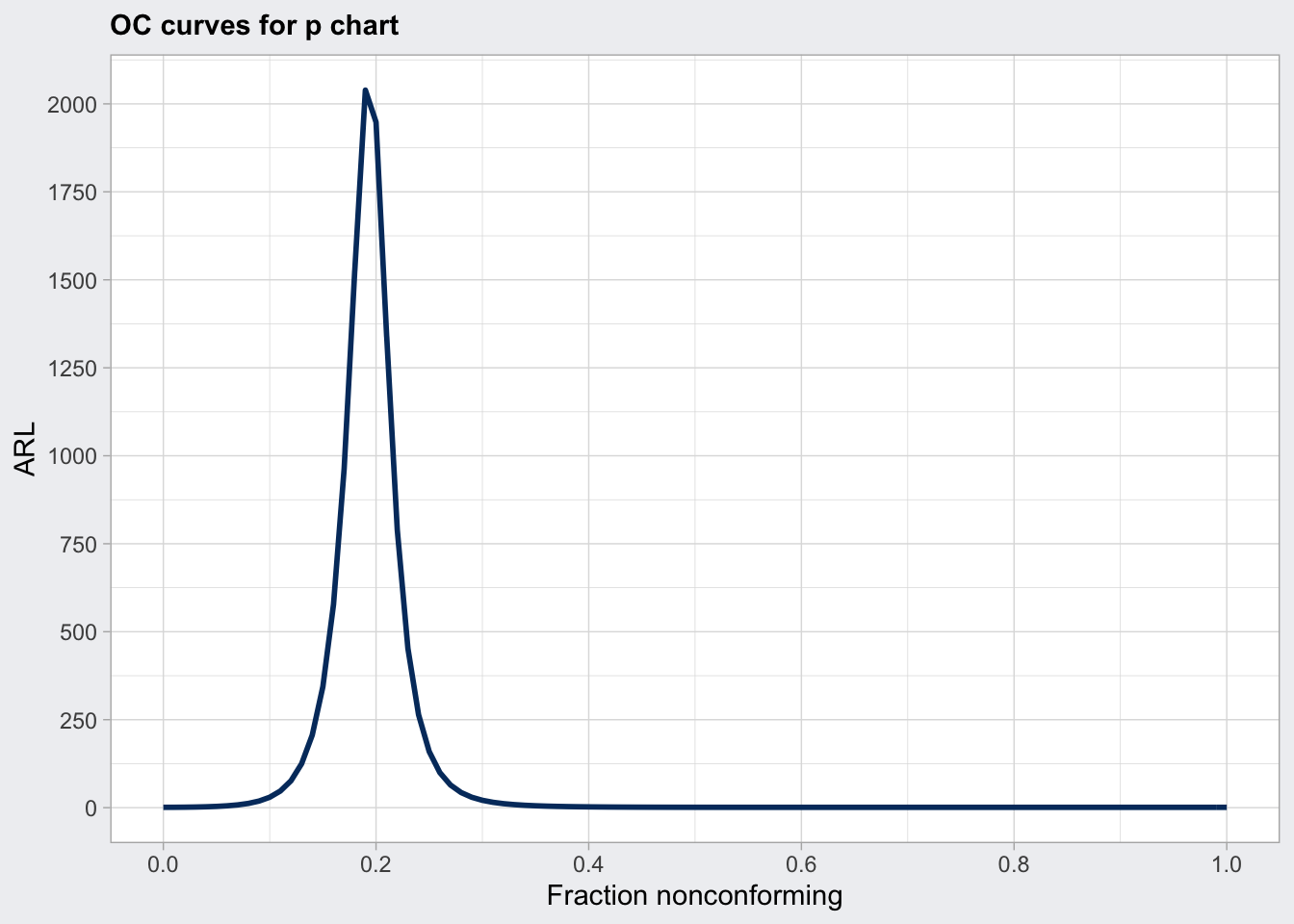``````q6a <- qcc(
df_oj\$D[df_oj\$trial],
df_oj\$size[df_oj\$trial],
type = "np",
newdata = df_oj\$D[! df_oj\$trial],
newsizes = df_oj\$size[! df_oj\$trial],
rules = c(1, 2, 3, 4)
)

q6a``````
``````## ── Quality Control Chart ─────────────────────────
##
## Chart type                 = np
## Data (phase I)             = df_oj\$D[df_oj\$trial]
## Number of groups           = 30
## Group sample size          = 50
## Center of group statistics = 11.56667
## Standard deviation         = 2.981763
##
## New data (phase II)        = df_oj\$D[!df_oj\$trial]
## Number of groups           = 24
## Group sample size          = 50
##
## Control limits at nsigmas  = 3
##       LCL      UCL
##  2.621377 20.51196``````
``plot(q6a)``### c chart

• for count of events that cannot be attributed to a sample size
• examples: nonconformities per day, defects per m^2 of fabric or flaws per metal plate
• unit should stay constant
``````library(SixSigma)

ss.data.thickness2 %>%
``````##    day shift thickness ushift flaws
## 1    1     1     0.713    1.1     9
## 2    1     1     0.776    1.1    NA
## 3    1     1     0.743    1.1    NA
## 4    1     1     0.713    1.1    NA
## 5    1     1     0.747    1.1    NA
## 6    1     1     0.753    1.1    NA
## 7    1     2     0.749    1.2     2
## 8    1     2     0.726    1.2     7
## 9    1     2     0.774    1.2     9
## 10   1     2     0.744    1.2    NA
## 11   1     2     0.718    1.2    NA
## 12   1     2     0.677    1.2    NA
## 13   2     1     0.778    2.1     7
## 14   2     1     0.802    2.1    NA
## 15   2     1     0.798    2.1    NA``````
``````# remove uninspected items
df_flaws <- ss.data.thickness2 %>%
as_tibble() %>%
filter(! is.na(flaws))

df_flaws``````
``````## # A tibble: 23 x 5
##    day   shift thickness ushift flaws
##    <fct> <fct>     <dbl> <chr>  <int>
##  1 1     1         0.713 1.1        9
##  2 1     2         0.749 1.2        2
##  3 1     2         0.726 1.2        7
##  4 1     2         0.774 1.2        9
##  5 2     1         0.778 2.1        7
##  6 2     2         0.78  2.2        4
##  7 2     2         0.729 2.2        6
##  8 2     2         0.793 2.2        9
##  9 3     1         0.775 3.1        9
## 10 3     2         0.727 3.2        4
## # … with 13 more rows``````
``````q7 <- qcc(
df_flaws\$flaws,
type = "c",
rules = c(1, 2, 3, 4)
)

q7``````
``````## ── Quality Control Chart ─────────────────────────
##
## Chart type                 = c
## Data (phase I)             = df_flaws\$flaws
## Number of groups           = 23
## Group sample size          = 1
## Center of group statistics = 5.26087
## Standard deviation         = 2.293659
##
## Control limits at nsigmas  = 3
##  LCL      UCL
##    0 12.14185``````
``plot(q7)````````q7_oc <- qcc::ocCurves(q7)

q7_oc``````
``````## ── Operating Characteristic Curves ───────────────
##
## Chart type                 = c
## Prob. type II error (beta) =
##        [,1]
## 0.00  1e+00
## 1.00  1e+00
## 2.00  1e+00
## :
## 28.00 6e-04
## 29.00 3e-04
## Average run length (ARL)   =
##              [,1]
## 0.00          Inf
## 1.00  15723815904
## 2.00      4822834
## :
## 28.00           1
## 29.00           1``````
``````plot(q7_oc, what = "ARL") +
coord_cartesian(ylim = c(0, 10))``````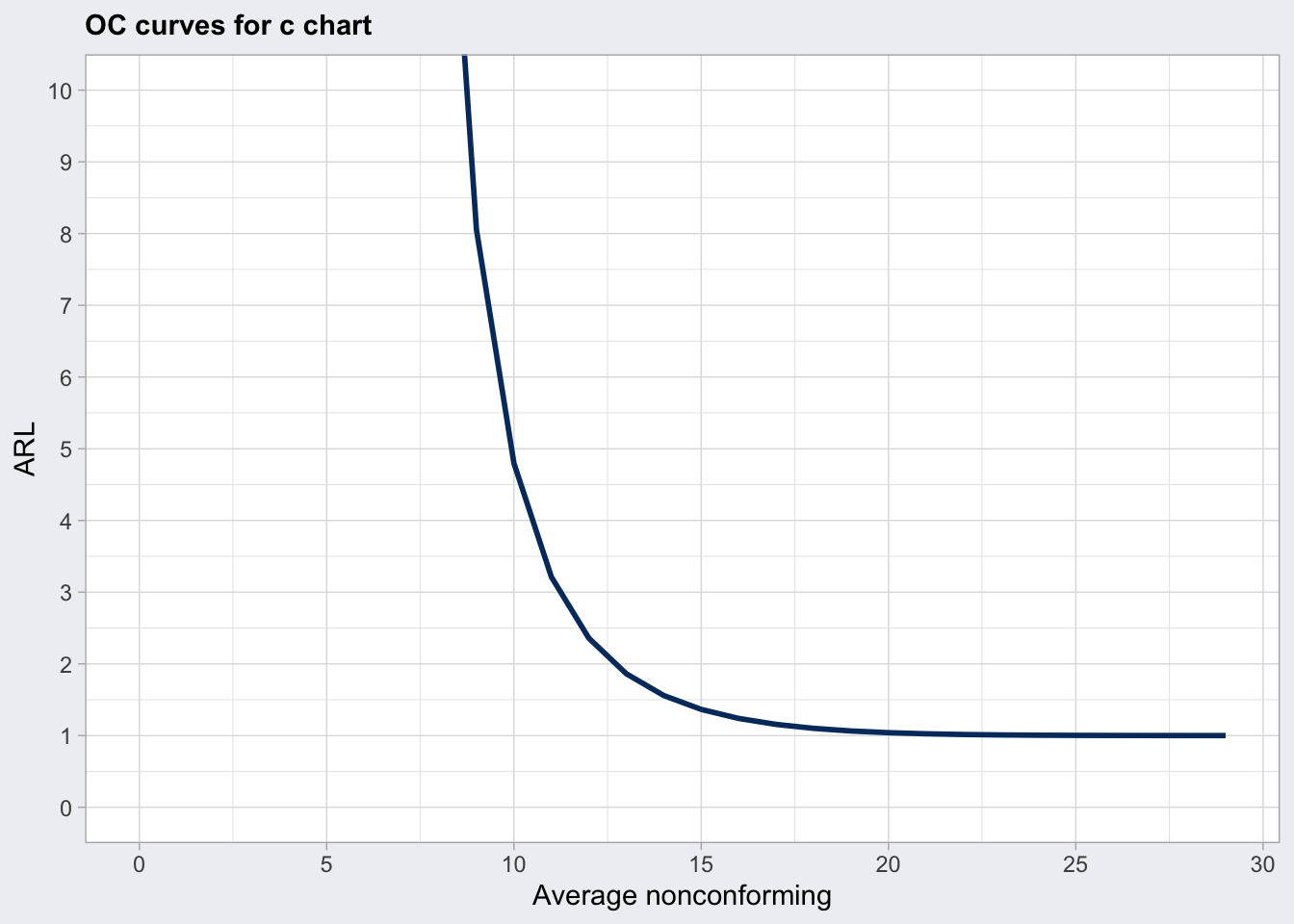### u chart

• average number of defects from a varying number of samples
• for example: 18 flaws from 3 metal plates, then 3 flaws in 5 metal plates
``````df_gr_flaws <- df_flaws %>%
group_by(ushift) %>%
summarise(n_plates_per_shift = n(),
n_flaws_per_shift = sum(flaws))

df_gr_flaws``````
``````## # A tibble: 14 x 3
##    ushift n_plates_per_shift n_flaws_per_shift
##    <chr>               <int>             <int>
##  1 1.1                     1                 9
##  2 1.2                     3                18
##  3 2.1                     1                 7
##  4 2.2                     3                19
##  5 3.1                     1                 9
##  6 3.2                     1                 4
##  7 4.1                     2                 6
##  8 4.2                     1                 4
##  9 5.1                     1                 5
## 10 5.2                     2                13
## 11 6.1                     2                 5
## 12 6.2                     1                 4
## 13 7.1                     1                 7
## 14 7.2                     3                11``````
``````qu8 <- qcc(
df_gr_flaws\$n_flaws_per_shift,
type = "u",
sizes = df_gr_flaws\$n_plates_per_shift,
rules = c(1, 2, 3, 4)
)

qu8``````
``````## ── Quality Control Chart ─────────────────────────
##
## Chart type                 = u
## Data (phase I)             = df_gr_flaws\$n_flaws_per_shift
## Number of groups           = 14
## Group sample sizes         =
##   sizes  1 2 3
##   counts 8 3 3
## Center of group statistics = 5.26087
## Standard deviation         = 2.293659
##
## Control limits at nsigmas  = 3
##        LCL       UCL
##   0.000000 12.141845
##   1.288136  9.233603
## :
##   1.288136  9.233603``````
``plot(qu8)``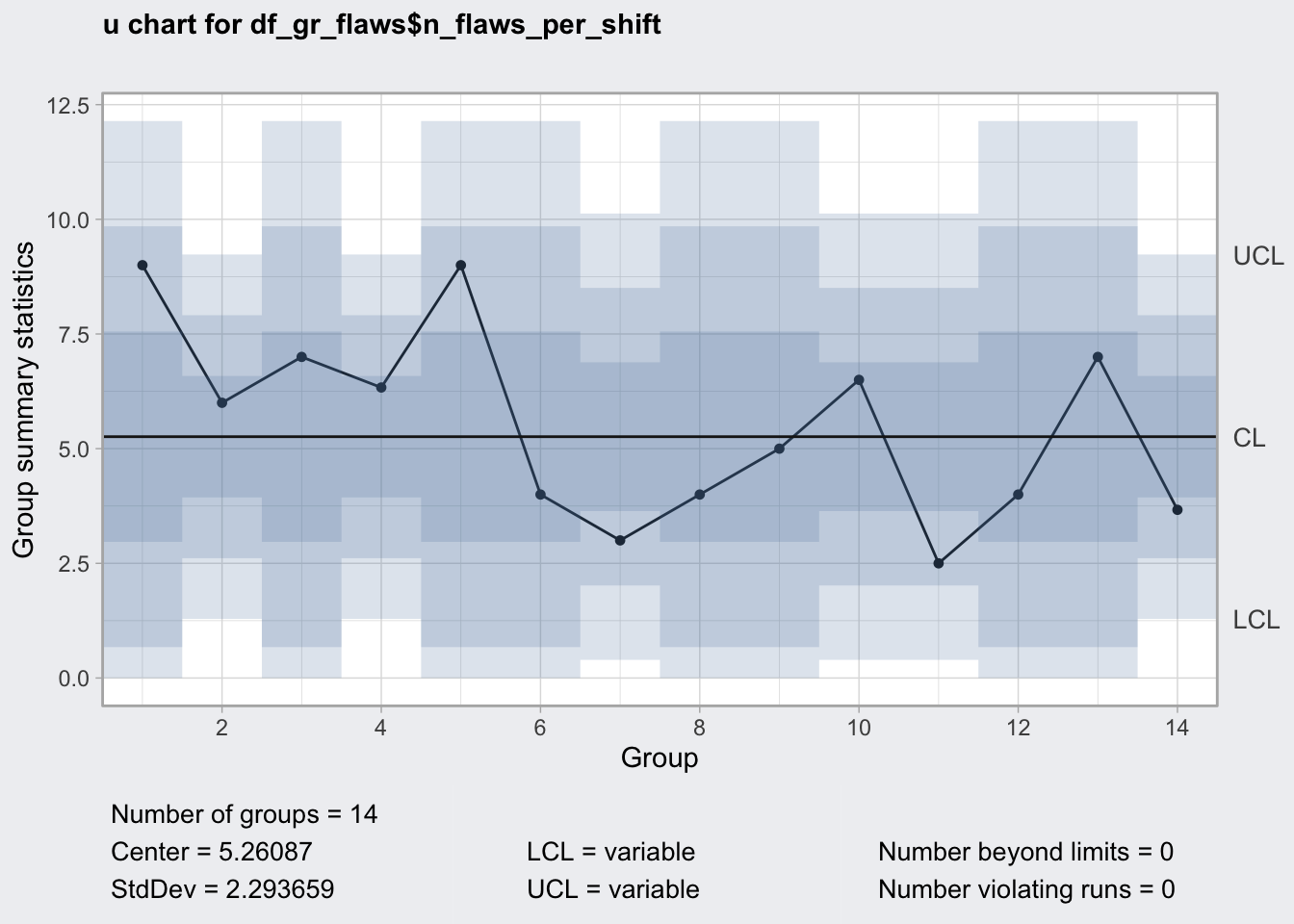``````# OC curves need constant sample sizes
# qu8_oc <- qcc::ocCurves(qu8)``````

## Capability/Performance Indices

• measure whether consumer specified upper and lower specification limits (LSL/USL) are compatible with process control limits (LCL/UCL)

• performance measures long-term variability and capacity short-term variability

• C = (USL - LSL) / 6sigma

• index below 1 is unsufficient, 1.33-167 is outstanding and 2 refers to six sigma quality

• adjusted index variations account for processes that are not perfectly centered

• Cp: capability index

• Cp_l: lower index

• Cp_u: upper index

• Cp_k: minimum of lower and upper index

• Cpm : Tagauchi index (controls for not centered processes)

``q1``
``````## ── Quality Control Chart ─────────────────────────
##
## Chart type                 = xbar
## Data (phase I)             = qcc_gr[1:25, ]
## Number of groups           = 25
## Group sample size          = 5
## Center of group statistics = 74.00118
## Standard deviation         = 0.009785039
##
## New data (phase II)        = qcc_gr[26:nrow(qcc_gr), ]
## Number of groups           = 15
## Group sample size          = 5
##
## Control limits at nsigmas  = 3
##       LCL     UCL
##  73.98805 74.0143``````
``````q1_pc <- qcc::processCapability(q1, spec.limits = c(73.975, 74.025))

q1_pc``````
``````## ── Process Capability Analysis ───────────────────
##
## Number of obs = 125          Target = 74
## Center        = 74.00118     LSL    = 73.975
## StdDev        = 0.009785039  USL    = 74.025
##
## Capability indices  Value   2.5%  97.5%
##               Cp    0.852  0.746  0.957
##               Cp_l  0.892  0.786  0.997
##               Cp_u  0.812  0.714  0.910
##               Cp_k  0.812  0.695  0.928
##               Cpm   0.846  0.740  0.951
##
## Exp<LSL 0.0037%   Obs<LSL 0.008%
## Exp>USL 0.0075%   Obs>USL 0.008%``````
``plot(q1_pc)``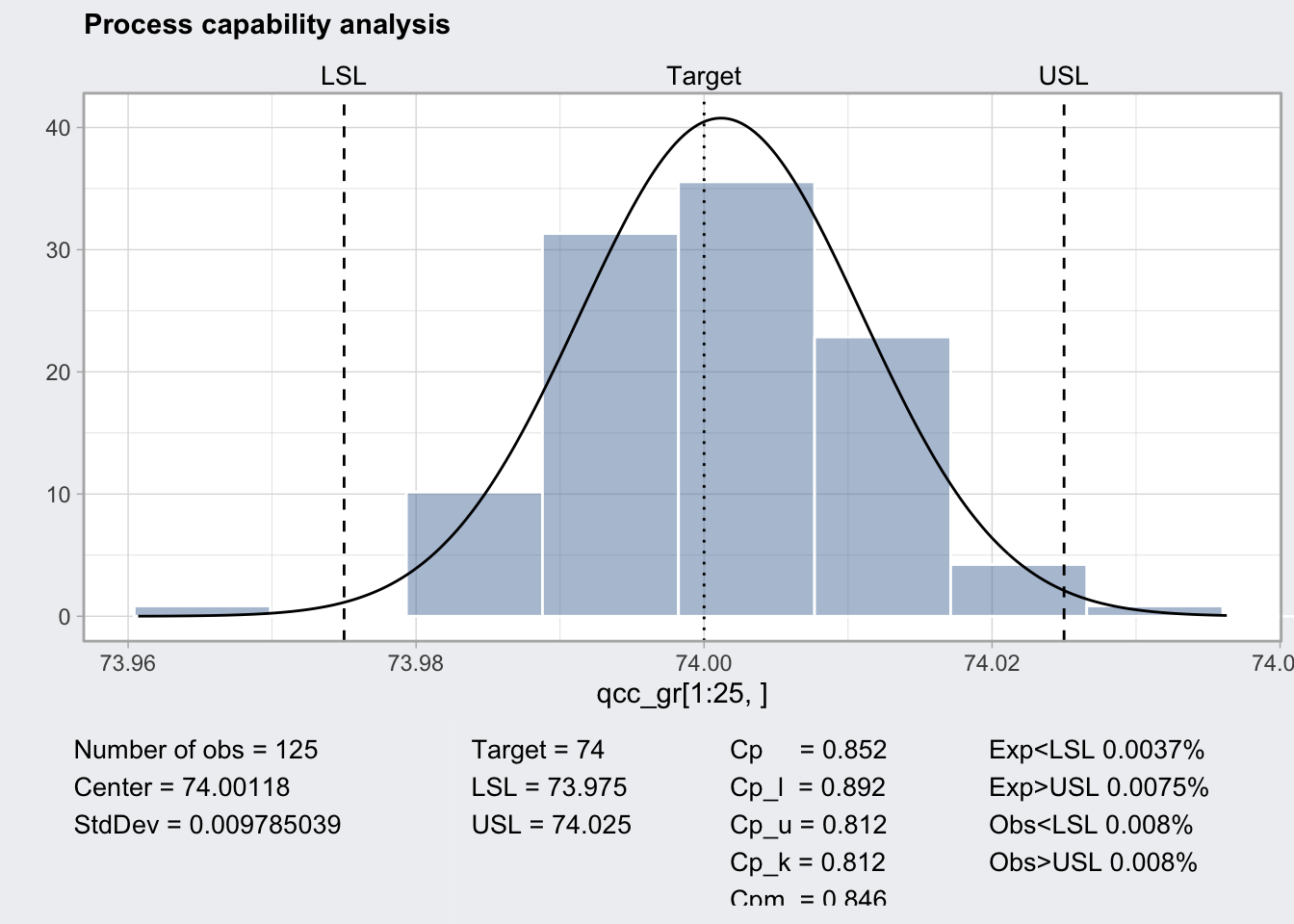``````q1_pc <- qcc::processCapability(q1, spec.limits = c(73.95, 74.05))

q1_pc``````
``````## ── Process Capability Analysis ───────────────────
##
## Number of obs = 125          Target = 74
## Center        = 74.00118     LSL    = 73.95
## StdDev        = 0.009785039  USL    = 74.05
##
## Capability indices  Value  2.5%  97.5%
##               Cp     1.70  1.49   1.91
##               Cp_l   1.74  1.55   1.93
##               Cp_u   1.66  1.48   1.84
##               Cp_k   1.66  1.45   1.88
##               Cpm    1.69  1.48   1.90
##
## Exp<LSL 0%    Obs<LSL 0%
## Exp>USL 0%    Obs>USL 0%``````
``plot(q1_pc)``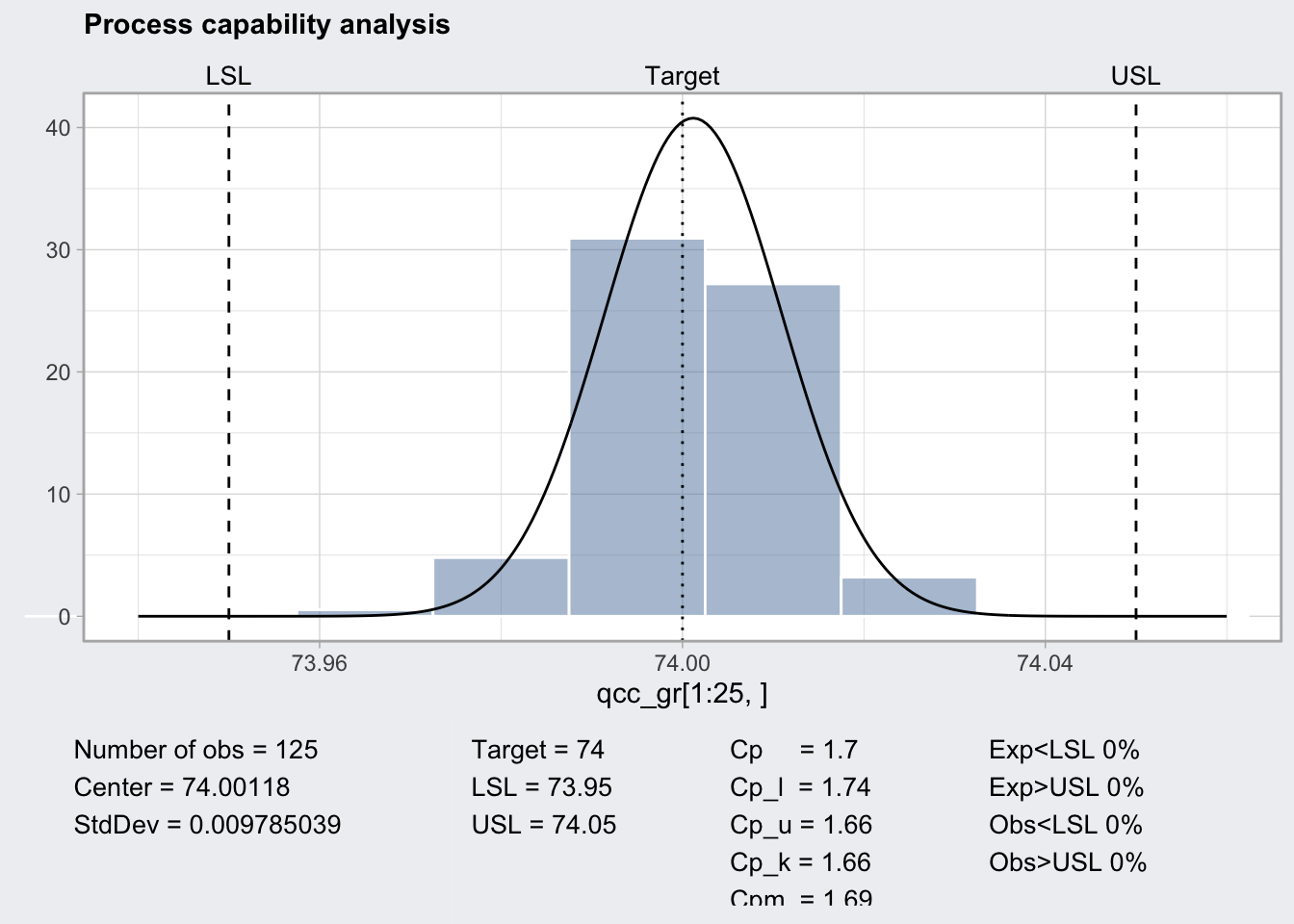## CUSUM Chart

• cumulative sum chart
• two lines one below zero and one above zero and a center which is the expected value.
• positive deviation of the process from the central value results in an uptick of both lines
• negative deviation of the process from the central value results in a down tick of both lines
• both lines never cross the central line
• supposedly more sensitive than regular control charts
``qcc_gr``
``````##      [,1]   [,2]   [,3]   [,4]   [,5]
## 1  74.030 74.002 74.019 73.992 74.008
## 2  73.995 73.992 74.001 74.011 74.004
## 3  73.988 74.024 74.021 74.005 74.002
## 4  74.002 73.996 73.993 74.015 74.009
## 5  73.992 74.007 74.015 73.989 74.014
## 6  74.009 73.994 73.997 73.985 73.993
## 7  73.995 74.006 73.994 74.000 74.005
## 8  73.985 74.003 73.993 74.015 73.988
## 9  74.008 73.995 74.009 74.005 74.004
## 10 73.998 74.000 73.990 74.007 73.995
## 11 73.994 73.998 73.994 73.995 73.990
## 12 74.004 74.000 74.007 74.000 73.996
## 13 73.983 74.002 73.998 73.997 74.012
## 14 74.006 73.967 73.994 74.000 73.984
## 15 74.012 74.014 73.998 73.999 74.007
## 16 74.000 73.984 74.005 73.998 73.996
## 17 73.994 74.012 73.986 74.005 74.007
## 18 74.006 74.010 74.018 74.003 74.000
## 19 73.984 74.002 74.003 74.005 73.997
## 20 74.000 74.010 74.013 74.020 74.003
## 21 73.988 74.001 74.009 74.005 73.996
## 22 74.004 73.999 73.990 74.006 74.009
## 23 74.010 73.989 73.990 74.009 74.014
## 24 74.015 74.008 73.993 74.000 74.010
## 25 73.982 73.984 73.995 74.017 74.013
## 26 74.012 74.015 74.030 73.986 74.000
## 27 73.995 74.010 73.990 74.015 74.001
## 28 73.987 73.999 73.985 74.000 73.990
## 29 74.008 74.010 74.003 73.991 74.006
## 30 74.003 74.000 74.001 73.986 73.997
## 31 73.994 74.003 74.015 74.020 74.004
## 32 74.008 74.002 74.018 73.995 74.005
## 33 74.001 74.004 73.990 73.996 73.998
## 34 74.015 74.000 74.016 74.025 74.000
## 35 74.030 74.005 74.000 74.016 74.012
## 36 74.001 73.990 73.995 74.010 74.024
## 37 74.015 74.020 74.024 74.005 74.019
## 38 74.035 74.010 74.012 74.015 74.026
## 39 74.017 74.013 74.036 74.025 74.026
## 40 74.010 74.005 74.029 74.000 74.020``````
``````q1 <- qcc(
qcc_gr[1:25, ],
type = "xbar",
newdata = qcc_gr[26:nrow(qcc_gr), ],
rules = c(1, 2, 3, 4)
)

plot(q1)``````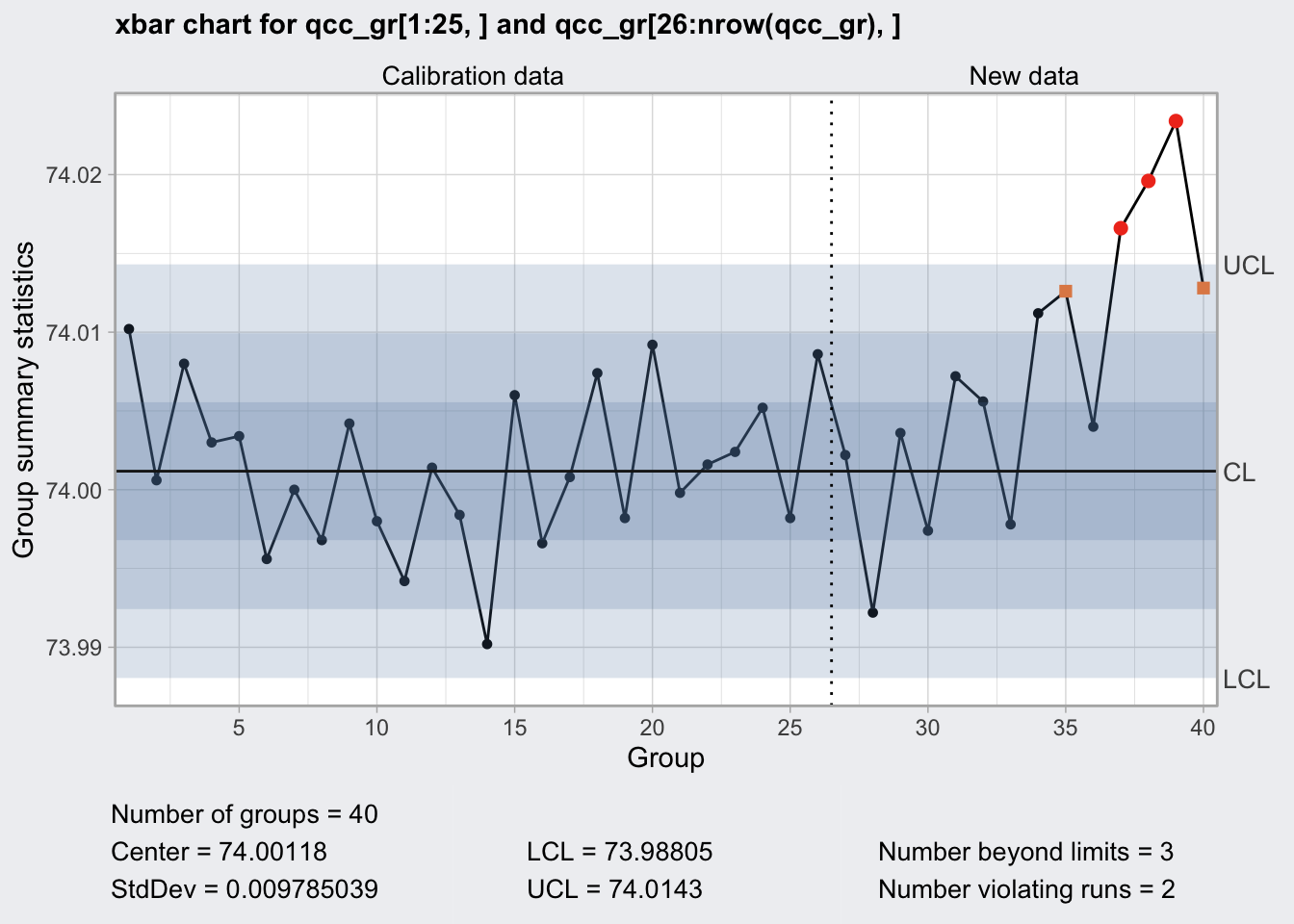``````q9 <- qcc::cusum(
qcc_gr[1:25, ],
newdata = qcc_gr[26:nrow(qcc_gr), ]
)

plot(q9)``````## EWMA Chart

• exponentially weighted moving average chart
• zj = gamma * xj + (1 - gamma) * zj-1
• if the smoothing parameter gamma is 0.2 a given value for x is 20% present value and 80% past values
• `+` indicates the actual values
• supposed to work well for not normal values
``````q10 <- qcc::ewma(
qcc_gr[1:25, ],
newdata = qcc_gr[26:nrow(qcc_gr), ]
)

plot(q10)``````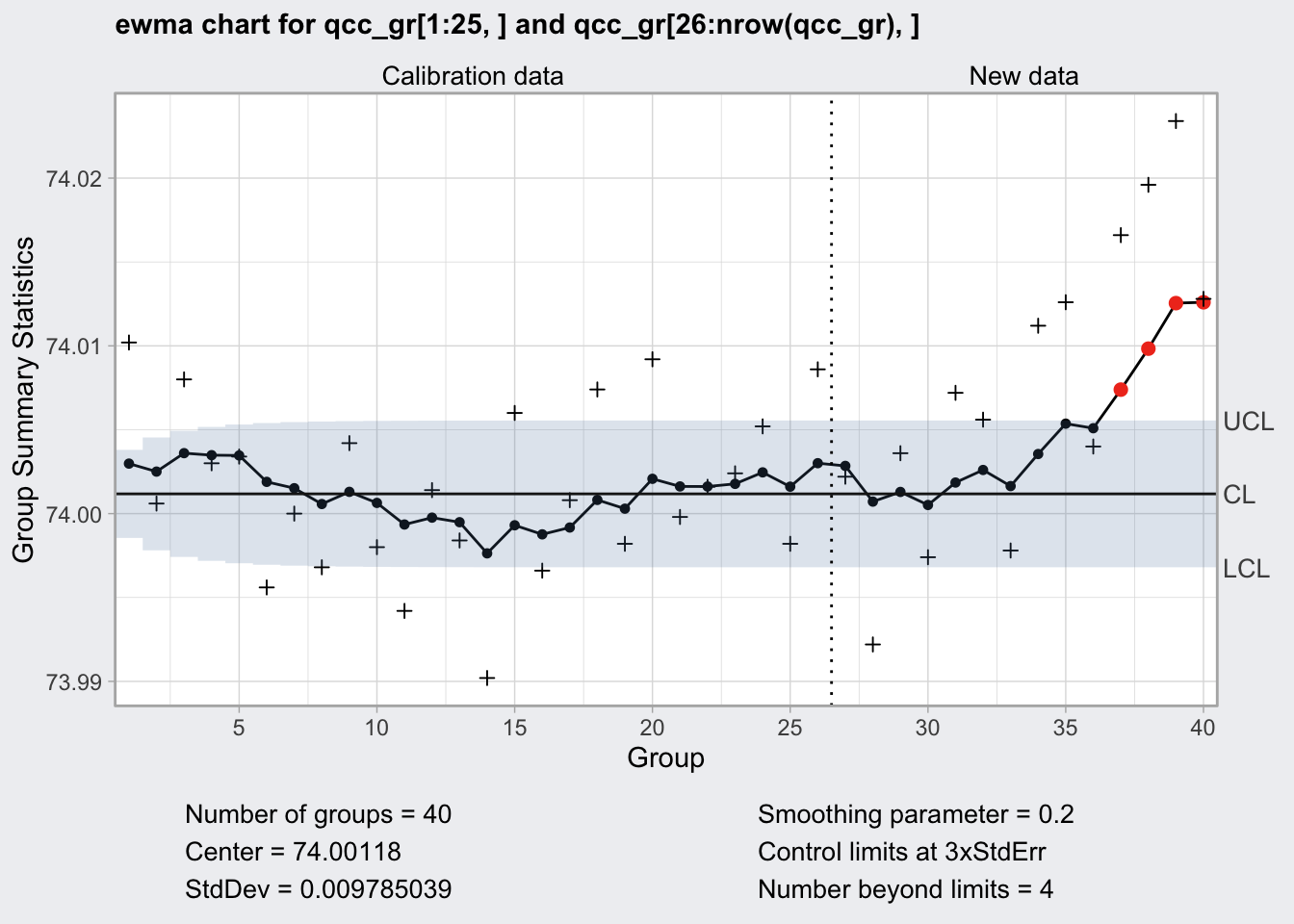# Sampling/Stratification

Naturally samples need to be representative of the population. If there are known subgroups it is important to adapt a stratified sampling strategy.

free ebook

## Attribute Acceptance Sampling

• attribute: defective / not defective
• producer wants a high probability of acceptance for a lot with a low defective fraction
• producers and consumer agree on acceptable quality level (AQL)
• alpha (producers risk) is the probability with which a lot that passes AQL is rejected
• consumer wants a high probability of rejection for a lot with a high defective ratio
• producers and consumer agree on lot tolerance percent defective (LTPD)
• beta (consumers risk) is the probability with which a lot that does not pass LTPD is accepted

Requirements:

• AQL: 0.06
• alpha: 0.05
• LTPD: 0.16
• beta: 0.10
``````library(AcceptanceSampling)

plan <- AcceptanceSampling::find.plan(
PRP = c(0.06, 0.95),
CRP = c(0.16, 0.10)
)

plan``````
``````## \$n
##  79
##
## \$c
##  8
##
## \$r
##  9``````
``````oc <- AcceptanceSampling::OC2c(plan\$n, plan\$c, plan\$r)

plot(oc, xlim = c(0, 0.25))``````take a sample of 79 and reject when 9 items are defective

## Variable Acceptance Sampling

specify:

• upper specification limit (USL)

and or:

• lower specification limit (LSL)
``````plan2 <- AcceptanceSampling::find.plan(
PRP = c(0.06, 0.95),
CRP = c(0.16, 0.10),
type = "normal",
s.type = "known"
)

plan2``````
``````## \$n
##  28
##
## \$k
##  1.243925
##
## \$s.type
##  "known"``````

proceed:

• take a sample of 28
• compute the mean
• compute LSL/USL - mean(sample) / sd
• compare accept if value above is greater 1.2439255
``````plan3 <- AcceptanceSampling::find.plan(
PRP = c(0.06, 0.95),
CRP = c(0.16, 0.10),
type = "normal",
s.type = "unknown"
)

plan3``````
``````## \$n
##  49
##
## \$k
##  1.245424
##
## \$s.type
##  "unknown"``````

proceed:

• take a sample of 49
• compute the mean
• compute LSL/USL - mean(sample) / sd(sample)
• compare accept if value above is greater 1.2454244

Note that sample size has increased because we do not know population sd and need to estimate it from the sample.